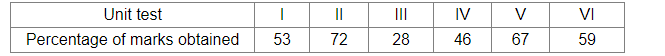# The percentages of marks obtained by a student in six unit tests are given below.

Question:

The percentages of marks obtained by a student in six unit tests are given below.A unit test is selected at random. What is the probability that the student gets more than 60% marks in the test?

Solution:

Total number of unit tests = 6
Number of tests in which the student scored more than 60% marks = 2

Let E be the event that he got more than 60% marks in the unit tests.Then,

required probability $=P(E)=\frac{\text { Number of unit tests in which he got more than } 60 \% \text { marks }}{\text { Total number of unit tests }}=\frac{2}{6}=\frac{1}{3}$